Kushwaha S. An Efficient Filtering Approach for Speckle Reduction in Ultrasound Images. Biomed Pharmacol J 2017;10(3).
Manuscript received on :June 02, 2017
Manuscript accepted on :July 11, 2017
Published online on: --
Plagiarism Check: Yes
How to Cite    |   Publication History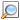Views: (Visited 526 times, 1 visits today)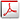PDF Downloads: 562
##### An Efficient Filtering Approach for Speckle Reduction in Ultrasound Images

Sumit Kushwaha

Electronics Engineering Department, Kamla Nehru Institute of Technology, Sultanpur, India.

Corresponding Author E-mail: sumit.kushwaha1@gmail.com

Abstract:

Ultrasound (US) imaging is a valuable imaging technique for clinical diagnosis. It is non-invasive in nature and imaging the internal structure of the body to identify the probabilistic diseases or, abnormalities in tissues behavior. However, inherent response of speckle noise in US images limit the fine and edge details which affect the contrast resolution. This makes clinical diagnosis more difficult.In this paper, we proposed a non-linear anisotropic diffusion filtering for speckle reduction approach based on non-linear progression partial differential equation (PDE). For analysis purpose, we have considered the set of eight-real clinical B-Mode US images of human liver from different patient. These real US images are used for quantitative analysis. We compare the performance of four speckle reduction filters as Perona-Malik Filter, LEE Filter, FROST Filter, ADMBSS Filter with our proposed filter in terms of peak signal to noise ratio (PSNR) value performance index under various noise variance selection Parmenter. Finally, we see that our proposed approach preserves the clinical details in US images and minimizing the noise level. Results for set of eight US images shows that our proposed filtering approach is more efficient for speckle noise reduction in comparison to other discussed filters in term of higher PSNR value (dB).

Keywords:

Diffusion filter; MATLAB;PDE; PSNR;Ultrasound;

Download this article as: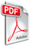Copy the following to cite this article: Kushwaha S. An Efficient Filtering Approach for Speckle Reduction in Ultrasound Images. Biomed Pharmacol J 2017;10(3).
 Copy the following to cite this URL: Kushwaha S. An Efficient Filtering Approach for Speckle Reduction in Ultrasound Images. Biomed Pharmacol J 2017;10(3). Available from: http://biomedpharmajournal.org/?p=16002

Introduction

Medical Ultrasound (US) Imaging technique is a non-ionizing radiations imaging modality that enables real time diagnosis treatment. This technique has non-invasive nature. It is widely used in medical field for diagnosis, patient routine check-ups for good health, and more and more use in surgeries and intrusions as a supervision modality. This inferior image quality is a challenging issue in US imaging as compared to other imaging modalities. The degree of degradation in US image quality can be highly varying and it depends on patients to patients. Sometimes it is an important challenge to imaging desired structures in particular pose of a fatty patient.1,2 US images suffers from numerous acoustic imaging objects including resonance, deviations and effect of speckle noise. In this paper, we focused on minimization of speckle noise effect in US images. Speckle noise effect seems like a granular texture effect on the US image. It is an inherent response of the backscattered interfering signal from the desired interrogated medium.3 In fact, an accurate description of the speckle noise pattern statistics is still an active research area which involves complex analytical modelling. Speckle noise behavior statistics may be characterized into different modules.4 Speckle noise effect is spatially correlated with correlation length which is calculated by the autocorrelation of the point spread function (PSF). In various US imaging cases, need for depth of diffusion leads to important speckle correlation lengths.

Earlier speckle reduction LEE filter5 and FROST filter6 were believed that speckle noise has additive and multiplication noise component in it. These filters are suitable for speckle reduction by minimizing mean squared error (MSE). These filter statistics are based estimation in local windows. The limitations with these filters are that it smoothed the image near structured and edges region. Perona and Malik filter7 is firstly adopted the anisotropic diffusion technique for speckle noise reduction in US Images. This filter avoids the unnecessary smoothing related with linear diffusion techniques, but not preserve edges details. A recent speckle reduction method ADMBSS filter8 proposes a memory based on speckle statistics filtering. This simple technique aims to apply memory mechanism as delay differential equation (DDE) for the diffusion tensor. The behavior of this memory mechanism follows the statistics of the tissues and preserves the clinical details in US images.

Finally, the main challenges with US filtering techniques are preserving the relevant clinical details and avoiding over filtering problem. Keep in mind these limitations and challenges of state-of-the-art filters, we proposed an efficient anisotropic diffusion filter for speckle reduction in US images. Our proposed filter gives better result for experiment with eight real US test images of different patients. This is the novelty of our proposed work.

Related Studies

Lee Filter For Speckle

The Lee filter reduces the speckle noise by applying a spatial filter to each pixel in an image, which filters the data based on local statistics calculated within a square window. The value of the center pixel is replaced by a value calculated using the neighboring pixels.4,5 This pointwise linear filter is based on the Minimum Mean Square Error (MMSE), and produced speckle noise free image based on the following equation (value of filtered pixel):where,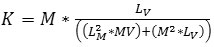andand

K= weight function,

Pc—Center pixel value of kernel/window (Median value)

Lm—Local mean of filter window

Lv—Local variance of filter window

M—Multiplicative noise mean (Default value: 1)

MV—Multiplicative noise variance (Default value: 0.25)

N Looks—Number of looks (Specifies the number of looks of the image. This is used to calculate the Multiplicative noise variance and control the amount of smoothing applied to the image. Using a smaller value for the Number of Looks leads to more smoothing, and a larger value preserves more image features.9)

The N Looks for a homogeneous region of an image is the ratio between the mean squared to the variance. The Looks is defined as follows: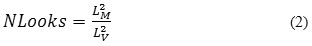The local mean Lof filter window is defined as: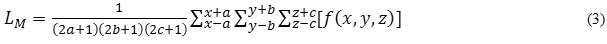Similarly, the local variance Lv of the filter window is defined as: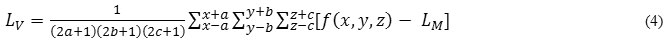From eq. (4), if value of Lis negative, in that case we have a very homogeneous area, Lshould be set to zero. Then estimateis given by the local mean LIf value of Lis very high, this indicates a very high contrast region (or, an edge presence) and=f (x, y, z). These extreme cases are in accordance with the Bayesian approach that is adopted in this linear MMSE filter.5

Frost Filter For Speckle

The FROST filter is used to design an adaptive filter algorithm to reduce speckle noise in spatial domain and computationally very efficient. This filter preserves the important features of image at the edges. It is a MMSE convolutional filter for speckle reduction. The Frost filter is an exponentially damped circularly symmetric filter that uses local statistics within individual filter windows. The pixel being filtered is replaced with a value calculated based on the distance from the filter center, the damping factor, and the local variance. The Frost filter requires a damping factor (define the extent of smoothing). The Damping Factor value defines the extent of exponential damping. The smaller the value is, the better the smoothing ability and filter performance. After application of the Frost filter, the denoised images show better sharpness at the edges.6,10,11 The algorithm used in the implementation of the Frost filter is as follows:

From eq. (1):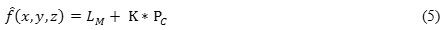where,

K = e-(B*S),

where,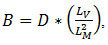and

S—Absolute value of the pixel distance from the center pixel to its neighbors in the filter window,

D—Exponential damping factor (Default value:1)

The factor D is chosen such that when in a homogeneous region, B approaches zero, yielding the mean filter output; at an edge B becomes so large that filtering is inhibited completely.12

Perona Malik Filter

Perona Malik filter is a classical diffusion filter technique for speckle reduction in US images. This diffusion filter is a linear and space invariant transformation of the original image. The resulting image in this filter obtained by convolution between the images and an isotropic Gaussian filter.13 Perona and Malik7 have given a name to their filter called anisotropic diffusion filter. This diffusion filter technique typically looks like the process that creates a scale space not a diffusion tensor, where an image generates a parameterized family of successively more and more blurred images based on a diffusion process.Diffusion is a physical process to create equilibrium concentration differences without destroying or, creating body mass. The Perona Malik filter is based on the equation: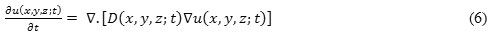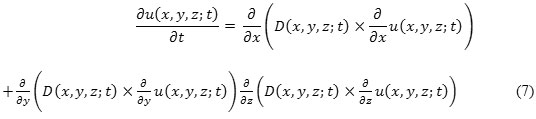with initial condition uo (x, y, z) = u (x, y,z: t =0) which is noisy image/input image. u (x, y, z: t) is the output image. D (x, y, z: t) is diffusion coefficient, known as symmetric positive definite tensor. D (x, y, z: t) also controls the rate of the diffusion. D (x, y, z: t) depends on local structure of  u (x, y,z: t) (if D is constant, then filter is isotropic diffusion filter and if D is not constant, then filter is anisotropic diffusion filter) and ∇. and  ∇ denote the divergence operator and gradient operator, respectively, u(x, y, z)  is the initial image, i.e. noisy image, t is temporal variable. Eq. (7), Linear Anisotropic Diffusion (LAD), is an elliptic Partial Differential Equation (PDE).14

By using finite difference method, eq. (7) given as:which is expressed as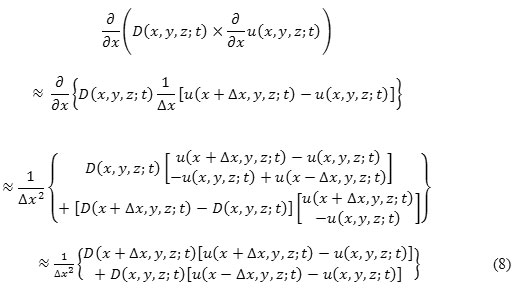where “≈” shows that the R.H.S. part of the equation is the difference approximation of the L.H.S. part.

Similarly, we have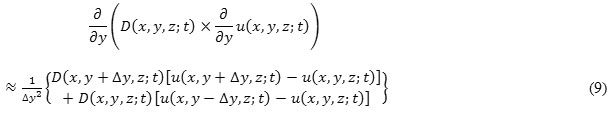and,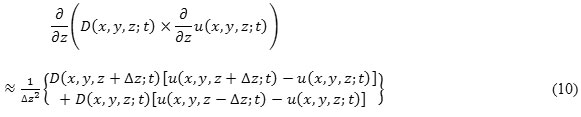All the values of eq. (8), eq. (9), and eq. (10) inserting in eq. (7) to obtain difference approximation ofPu Δx = 1, Δy = 1, and Δz = 1we get: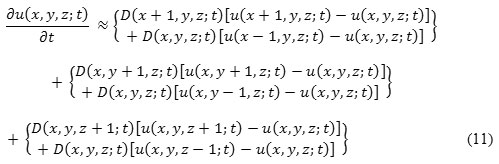So, obtaining discrete realization of anisotropic diffusion filter for u (x, y,z: t) image from eq. (11):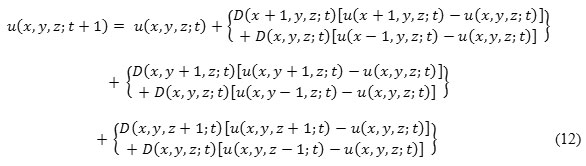In eq. (12), we can see that the major problem is selection of diffusion coefficient D (x, y,z: t) in anisotropic diffusion filter.

Diffusion coefficient D (x, y,z: t) is calculated as15: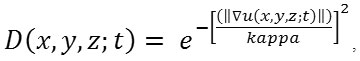when diffusion occurs across the boundaries and applies it in homogeneous areas. and,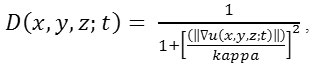When diffusion occurs near the boundaries and applies it in homogeneous areas.

Where, kappa is the gradient modulus threshold that controls the diffusion coefficient. Also, kappa controls the sensitivity near the edges and chosen experimentally or as a function of the noise in the image (kappa > 0).

Anisotropic Diffusion for Memory Based on Speckle Statistics (ADMBSS) Filter

ADMBSS filter8is to eliminate the effect of gradient information due to the lack of contours and low contrast of US images with objective of preservation of relevant clinical details in interest region using probabilistic-driven selective memory mechanism filtering. This filter is adapted to the US medical imaging context.9 For preserving clinical information in US images, G. Ramos-Llordénet. al.8 consider two different methods for tissue classification. 1st selective diffusion tensor method and 2nd probability driven memory methods in region of interest for tissue classification

A selective filtering tensor operator 0 (x, y, z: t) used as transformation of the instantaneous diffusion tensor D (x, y, z: t) at location (x, y, z) into a null tensor for suitable tissue classification and preservation. In this context, p (x, y, z: t) = 1 – p (x, y,z: t) for the probability of the tissue regions and p (x, y, z: t) for the probability of the non-tissue (meaningless) regions. For this selective behavior, the diffusion tensor D (x, y, z: t) is multiplied by its Eigenvalues by  p (x, y, z: t). Memory mechanism used, to know the anisotropic diffusion direction satisfy the condition that  p (x, y, z: t) → 0, so τ (x, y, z)  p (x, y, z: t) → ∞ . Memory mechanism will be disable if  p (x, y, z: t) → 1, so that τ (x, y, z)  p (x, y, z: t) → 0.  So the new reconstruct diffusion tensor by using expansion of outer product: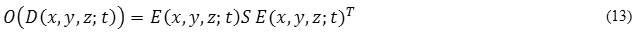where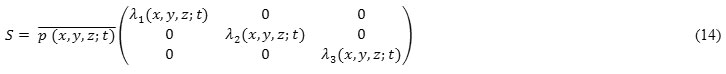and,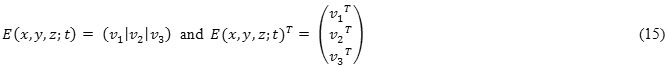Preserving pathway of the time dependent probability for getting more robust characterization than obtained from instantaneous probability, p (x, y, z: t),  tensor operator O (x, y, z: t) is not directly applied to  L (x, y, z: t). This p (x, y, z: t) provides more robust characterization than p (x, y, z: t).

Now, delay differential equation (DDE), with same initial and periodic condition as  and τ (x, y, z) is the spatial dependence of  τ, will be: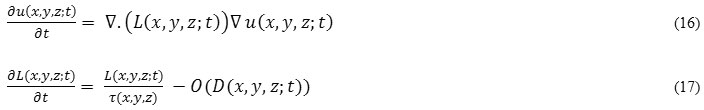Where, L (x, y, z: t) is the diffusion tensor matrix at point (x, y, z) and time t. Integrate eq. (52), we get:To turn ON/OFF the memory mechanism, spatial dependence τ (x, y, z) should satisfied the minimum conditions that τ (x, y, z): [0,1] → (0, ∞). The anisotropic diffusion flux  F (x, y, z: t) = L (x, y, z: t) ∇ u (x, y, z: t), then from eq. (18):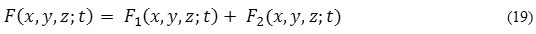Where filtered diffusion tensor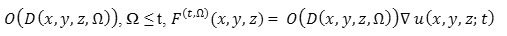and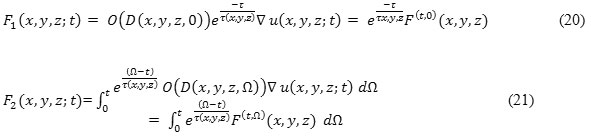Proposed Non-Linear Anisotropic Diffusion Filter

Most of the diffusion filters are simply modifications of perona-malik filter  where D is constant (scalar coefficient based on gradient of the image D ∇ u (x, y, z: t) which avoids diffusion near the boundaries and applies it in homogeneous areas). Here, we propose an efficient non-linear anisotropic diffusion for speckle reduction filtering approach based on non-linear progression of PDE. This proposed filter selects finite power intensity image uo (x, y, z) and having none zero-valued intensities over the image domain Ω. Here,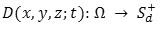is a given field of symmetric positive definite diffusion tensors where Ω is an open region of3 and ∂Ω is boundary of Ω. Eigenvectors  { v1 , v2 , v3 ,of these tensors define preferential diffusion directions, and the Eigenvalues their corresponding coefficients. Evolution rule eq. (6) is complemented with an initial condition u (x, y, z; 0) = uo (x, y, zat time t = 0. If  has pixels of vector type, then their components are treated independently.5,16 We get the output image u (x, y, z; tby following PDE: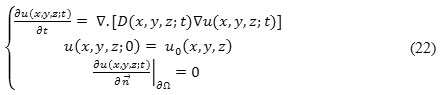where ∂Ω denotes the boundary of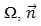is the outer normal to the ∂Ω, and D (x, y, z)  is coefficient of diffusion which is defined as a decreasing function of the instantaneous coefficient of variation.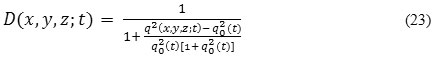or,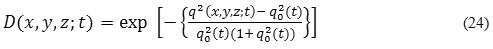In eq. (23) and (24), q (x, y, z; t) is the instantaneous coefficient of variation serves as the edge detector in speckled imagery, q(t) is the speckle scale function and is estimation parameter related to the coefficient of variation of noise. q (x, y, z; t) is determined by: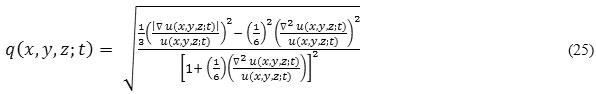In case of 2-D, instantaneous coefficient of variation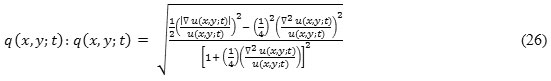Here, four stage iterative method can be used to solve mathematically. Let anisotropic diffusion time step ∇ t  and spatial step h in x, y, z directions respectively, then the discretization of time and space coordinates as t = n Δ t; n = 0, 1, 2, 3, … … and x = ih, y = jh, z = kh; i = 0,1,2,3, … … ., M – 1, j =0,1,2,3, … , N – 1, k = 0,1,2,3, … … . , k – 1 respectively, where Mh x Nh x kh is support image size.For mathematical applications, we choose h = 1, and Δ t = 0.05. Let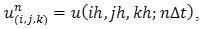then iterative method can be described as: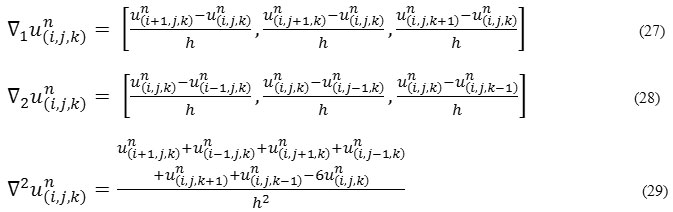Here, we are using symmetric boundary conditions. So: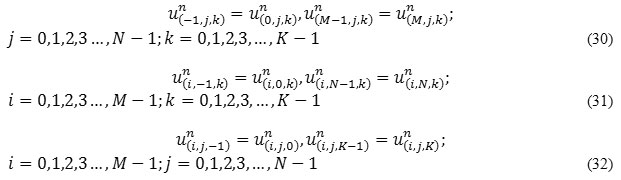Experiment and Discussion

In this experiment, we have considered the set of eight-real clinical B-Mode US images of human liver from different patient.17,18 These real US images are used for quantitative analysis. The size of these real clinical B-Mode US images of human liver is 256 x 256 x 24 (in pixel unit) in x, y, and z directions respectively. This data set is fed into the MATLAB19 platform for quantitative analysis. For measuring the all filters performance, we have used Peak Signal to Noise Ratio (PSNR) value (measured in dB).20 Higher PSNR value means higher level of image quality reconstruction. We have tested the performance of the filters (LEE Filter,5 FROST Filter,6 Perona Malik Filter,7 ADMBSS Filter8 and Proposed Filter) for all US images under different noise variance as 0.01, 0.04, 0.07, 0.1, and 0.5. All quantitative analysis results are represented in table form from Table I to VIII, and also shown in graphical form in figure 2. The output image of all filters under different noise variances are shown sequentially from figure 3 to figure 7. Now, we have seen that our proposed filter gives higher PSNR value under different noise variances in comparison to other discussed filters. This shows that our proposed filter is work efficiently for speckle noise reduction in US images under different noise variances.Figure 1: Experiment results obtain from these eight B-mode US images of human liver.   Click here to View figure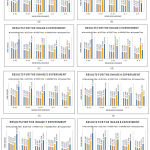Figure 2: The graphs are plotted for PSNR result values for different filters. These graphs show that our proposed filter is more efficient for removing Speckle noise.   Click here to View figureFigure 3: Noise Level Variance 0.01   Click here to View figure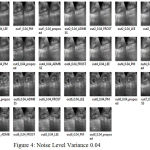Figure 4: Noise Level Variance 0.04   Click here to View figure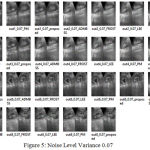Figure 5: Noise Level Variance 0.07   Click here to View figure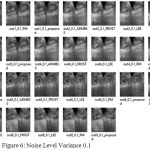Figure 6: Noise Level Variance 0.1   Click here to View figure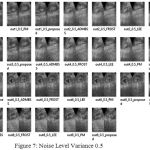Figure 7: Noise Level Variance 0.5   Click here to View figure

Table 1: Performance Comparison of Different Filters for Image 1

 PSNR(dB) RESULTS FOR THE IMAGE 1 EXPERIMENT Noise Level Perona Malik Filter LEE Filter FROST Filter ADMBSS Filter Proposed Filter 0.01 33.5863 30.7646 27.9208 33.0753 59.9532 0.04 26.8198 25.5545 24.1795 33.8953 52.0865 0.07 24.2403 23.0597 22.0432 43.5523 43.3213 0.1 22.8419 21.5929 20.7415 37.116 39.3994 0.5 18.6482 15.4397 15.0582 34.3762 28.4605

Table 2: Performance Comparison of Different Filters for Image 2

 PSNR(dB) RESULTS FOR THE IMAGE 2 EXPERIMENT Noise Level Perona Malik Filter LEE Filter FROST Filter ADMBSS Filter Proposed Filter 0.01 31.8765 29.6114 27.4193 38.7373 60.1176 0.04 25.3038 24.1023 23.0549 37.0798 46.9497 0.07 23.1179 21.8245 21.0142 54.1903 39.5293 0.1 21.8242 20.3137 19.6463 47.3763 36.3813 0.5 18.2999 14.5549 14.2363 42.1617 27.4346

Table 3: Performance Comparison of Different Filters for Image 3

 PSNR(dB) RESULTS FOR THE IMAGE 3 EXPERIMENT Noise Level Perona Malik Filter LEE Filter FROST Filter ADMBSS Filter Proposed Filter 0.01 33.6423 30.7505 27.9583 31.5686 60.4925 0.04 26.838 25.6038 24.1918 31.7607 51.1155 0.07 24.3578 23.1577 22.1389 44.7001 43.5445 0.1 22.8507 21.5427 20.7171 29.142 38.5877 0.5 18.6508 15.4617 15.0717 39.746 28.6693

Table 4: Performance Comparison of Different Filters for Image 4

 PSNR(dB) RESULTS FOR THE IMAGE 4 EXPERIMENT Noise Level Perona Malik Filter LEE Filter FROST Filter ADMBSS Filter Proposed Filter 0.01 31.8291 29.5409 27.3701 37.9569 61.7416 0.04 25.2852 24.0751 23.0358 34.5986 45.9795 0.07 23.1725 21.8448 21.0474 50.9217 39.4964 0.1 21.8462 20.3749 19.6957 41.2622 36.0276 0.5 18.2611 14.545 14.2376 43.3388 27.3917

Table 5: Performance Comparison of Different Filters for Image 5

 PSNR(dB) RESULTS FOR THE IMAGE 5 EXPERIMENT Noise Level Perona Malik Filter LEE Filter FROST Filter ADMBSS Filter Proposed Filter 0.01 32.7224 29.9306 27.1693 45.0071 59.175 0.04 27.2024 25.7441 24.1846 41.735 52.7133 0.07 24.9565 23.6137 22.4323 38.3478 44.5785 0.1 23.5763 22.1666 21.1989 40.7795 40.0525 0.5 19.3568 16.1321 15.705 42.24 29.3316

Table 6: Performance Comparison of Different Filters for Image 6

 PSNR(dB) RESULTS FOR THE IMAGE 6 EXPERIMENT Noise Level Perona Malik Filter LEE Filter FROST Filter ADMBSS Filter Proposed Filter 0.01 34.1398 31.1547 28.1883 32.4728 60.5218 0.04 25.2852 26.0935 24.6419 28.4759 53.269 0.07 24.9829 23.8935 22.6956 33.6521 44.4787 0.1 23.474 22.2108 21.3055 32.4591 40.083 0.5 18.9455 15.9552 15.5656 43.2921 29.074

Table 7: Performance Comparison of Different Filters for Image 7

 PSNR(dB) RESULTS FOR THE IMAGE 7 EXPERIMENT Noise Level Perona Malik Filter LEE Filter FROST Filter ADMBSS Filter Proposed Filter 0.01 33.7113 30.9191 28.3638 36.439 60.8786 0.04 27.2024 25.6571 24.4113 44.4852 49.149 0.07 24.6137 23.355 22.4096 34.3248 41.7057 0.1 23.2736 21.9426 21.1419 29.9298 38.9144 0.5 19.0164 15.8372 15.4541 18.3136 29.0295

Table 8: Performance Comparison of Different Filters for Image 8

 PSNR(dB) RESULTS FOR THE IMAGE 8 EXPERIMENT Noise Level Perona Malik Filter LEE Filter FROST Filter ADMBSS Filter Proposed Filter 0.01 31.4013 28.6532 26.1152 38.0811 62.3628 0.04 25.9988 24.4407 23.009 39.4908 49.7713 0.07 24.7752 22.3701 21.2703 40.2364 41.8029 0.1 22.447 20.9436 20.0514 26.1845 38.1168 0.5 18.6631 15.1269 14.7353 20.7533 28.1388

Conclusion

Speckle noise is inherent response in US images. Since it degraded the image quality and affecting fine and edge details. So, it is difficult task to see the clinical details in patient diagnosis. In this paper, we proposed a non-linear anisotropic diffusion filtering based speckle reduction approach based on non-linear progression PDE. This approach minimizes the speckle noise, preserves the clinical diagnosis details of patient. The experimental analysis tested on set of eight-real clinical B-Mode US images of human liver from different patientunder various noise variance selection Parmenter. We compare the performance of Perona-Malik Filter, LEE Filter, FROST Filter, ADMBSS Filter with our proposed non-linear anisotropic diffusion based speckle reduction filter.  We see that our proposed approach preserves the clinical details in US images and minimizing the noise level in term of higher PSNR value (dB). This is very helpful approach for radiologists/Doctors to accurate clinical diagnosis. Future works will include speckle reduction for more real time US images as well as in real time US imaging video.

References

1.  Destrempes F and  Cloutier G. A critical review and uniformized representation of statistical distributions modeling the ultrasound echo envelope.Ultrasound Med. Biol. 2010;36(7):1037–51.
CrossRef
2. Tay P. C.,  Garson C. D., Acton S. T and Hossack J. a. Ultrasound despeckling for contrast enhancement. IEEE Trans. Image Process. 2010;19(7):1847–60.
CrossRef
3. Rabbani H., Vafadust M.,  Abolmaesumi P and  Gazor S. Speckle noise reduction of medical ultrasound images in complex wavelet domain using mixture priors. IEEE Trans. Biomed. Eng. 2008;55(9):2152–60.
CrossRef
4. Krissian K.,  Westin C. F.,Kikinis R and Vosburgh K. G. Oriented speckle reducing anisotropic diffusion. IEEE Trans. Image Process. 2007;16(5):1412–1424.
CrossRef
5. Jean-Marie M., Jer^ome F., Risser L andTobji S. Anisotropic Diffusion in ITK.  arXiv: 1503.00992v1 [cs.CV]. 2015.
6.  Frost V.,Stiles J.,  Shanmugan K and Holzman J. A model for radar images and its application to adaptive digital filtering of multiplicative noise. IEEE Trans. PAMI. 1982;4(2):157–166.
CrossRef
7. Perona P and Malik J. Scale-space and edge detection using anisotropic diffusion. IEEE Trans. Pattern Anal. Mach. Intell. 1990;12(7):629–639.
CrossRef
8.  Ramos-Llordén G., Vegas-Sánchez-Ferrero G., Martin-Fernandez M., Alberola-López C and Aja-Fernández S. Anisotropic Diffusion Filter With Memory Based on Speckle Statistics for Ultrasound Images. in IEEE Transactions on Image Processing. 2015;24(1):345-358.
CrossRef
9.  Cottet G. H and Ayyadi M. E. A Volterra type model for image processing. IEEE Trans. Image Process. 1998;7(3):292–303.
CrossRef
10. Krissian K., Westin C. F., Kikinis R and Vosburgh K. G. Oriented speckle reducing anisotropic diffusion. IEEE Trans. Image Process. 2007;16(5):1412–1424.
CrossRef
11.  Yu Y and  Acton S. T. Speckle reducing anisotropic diffusion. IEEE Trans. Image Process. 2002;11(11):1260–1270.
CrossRef
12. Yu Y and Acton S. Edge detection in ultrasound imagery using the instantaneous coefficient of variation. IEEE Transactions on Image Processing. 2004;13(12):1640–1655.
CrossRef
13.  Weickert J.  Anisotropic Diffusion in Image Processing, Stuttgart, Germany: Teubner. 1998.
14. Krissian K.,  Westin C. F.,Kikinis R and Vosburgh K. G. Oriented speckle reducing anisotropic diffusion. IEEE Trans. Image Process. 2007;16(5):1412–1424.
CrossRef
15.  Aja-Fernandez S and Alberola-Lopez C. On the estimation of the coefficient of variation for anisotropic diffusion speckle filtering. IEEE Transactions on Image Processing in press.  2005;15(9):2694– 2701.
CrossRef
16.  Cottet G. H and Ayyadi M. E. A Volterra type model for image processing. IEEE Trans. Image Process.  1998;7(3):292–303.
CrossRef
17. Ultrasound Images Ultrasound images of diseases of the liver Accessed on 21st December. 2016. Available at http://www.ultrasound-images.com/liver/.
18. Online Medical Images, Ultrasound Images, Accessed on 21st December. 2016, Available at http://www.onlinemedicalimages.com/index.php/en/site-map.
19. MATLAB – Mathworks. The Language of Technical Computing tool, version R 2016, website: https://www.mathworks.com.
20. Peak Signal-to-Noise Ratio (PSNR) – MATLAB psnr – MathWorks India, available online at: http://in.mathworks.com/help/images/ref/psnr.html.
(Visited 526 times, 1 visits today)